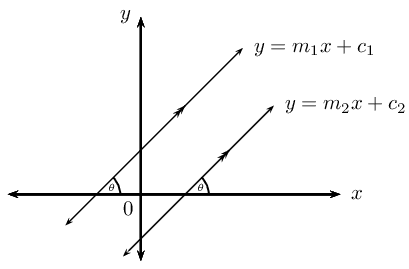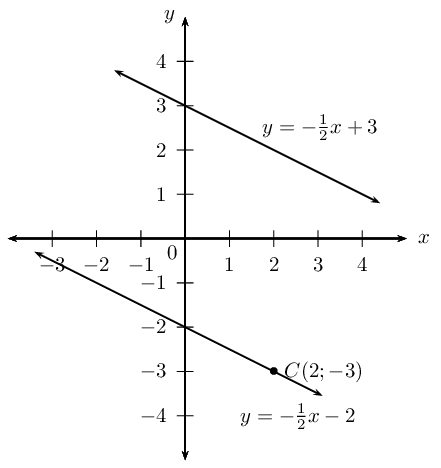Home Practice
For learners and parents For teachers and schools
Textbooks
Full catalogue
Pricing SupportLog in

We think you are located in United States. Is this correct?

# 4.4 Parallel lines

## Parallel lines

1. Draw a sketch of the line passing through the points $$P(-1;0)$$ and $$Q(1;4)$$ and the line passing through the points $$R(1;2)$$ and $$S(2;4)$$.
2. Label and measure $$\alpha$$ and $$\beta$$, the angles of inclination of straight lines $$PQ$$ and $$RS$$ respectively.
3. Describe the relationship between $$\alpha$$ and $$\beta$$.
4. “$$\alpha$$ and $$\beta$$ are alternate angles, therefore $$PQ \parallel RS$$.” Is this a true statement? If not, provide a correct statement.
5. Use your calculator to determine $$\tan \alpha$$ and $$\tan \beta$$.
6. Complete the sentence: $$\ldots \ldots$$ lines have $$\ldots \ldots$$ angles of inclination.
7. Determine the equations of the straight lines $$PQ$$ and $$RS$$.
8. What do you notice about $$m_{PQ}$$ and $$m_{RS}$$?
9. Complete the sentence: $$\ldots \ldots$$ lines have $$\ldots \ldots$$ gradients.

Another method of determining the equation of a straight line is to be given a point on the unknown line, $$\left({x}_{1};{y}_{1}\right)$$, and the equation of a line which is parallel to the unknown line.

Let the equation of the unknown line be $$y = m_1x + c_1$$ and the equation of the given line be $$y = m_2x + c_2$$.If the two lines are parallel then

$m_1 = m_2$

Important: when determining the gradient of a line using the coefficient of $$x$$, make sure the given equation is written in the gradient–intercept (standard) form.

$y=mx+c$

Substitute the value of $$m_2$$ and the given point $$\left({x}_{1};{y}_{1}\right)$$, into the gradient–intercept form of a straight line equation

$y - y_1 = m(x - x_1)$

and determine the equation of the unknown line.

## Worked example 11: Parallel lines

Determine the equation of the line that passes through the point $$(-1;1)$$ and is parallel to the line $$y - 2x + 1 = 0$$.

### Write the equation in gradient–intercept form

We write the given equation in gradient–intercept form and determine the value of $$m$$.

$y = 2x - 1$

We know that the two lines are parallel, therefore $$m_1 = m_2 = 2$$.

### Write down the gradient–point form of the straight line equation

$y - y_1 = m(x - x_1)$

Substitute $$m = 2$$

$y - y_1 = 2(x - x_1)$

Substitute the given point $$(-1;1)$$

\begin{align*} y - 1 & = 2(x - (-1)) \\ y - 1& = 2x + 2\\ y & = 2x + 2 + 1\\ &= 2x + 3 \end{align*}A sketch was not required, but it is always helpful and can be used to check answers.

The equation of the straight line is $$y = 2x + 3$$.

temp text

## Worked example 12: Parallel lines

Line $$AB$$ passes through the point $$A(0;3)$$ and has an angle of inclination of $$\text{153,4}$$$$\text{°}$$. Determine the equation of the line $$CD$$ which passes through the point $$C(2;-3)$$ and is parallel to $$AB$$.

### Use the given angle of inclination to determine the gradient

\begin{align*} m_{AB} & = \tan \theta \\ & = \tan \text{153,4}\text{°} \\ & = -\text{0,5} \end{align*}

### Parallel lines have equal gradients

Since we are given $$AB \parallel CD$$,

$m_{CD} = m_{AB} = -\text{0,5}$

### Write down the gradient–point form of a straight line equation

$y - y_1 = m(x - x_1)$

Substitute the gradient $$m_{CD} = -\text{0,5}$$.

$y - y_1 = -\frac{1}{2}(x - x_1)$

Substitute the given point $$(2;-3)$$.

\begin{align*} y - (-3) & = -\frac{1}{2}(x - 2) \\ y + 3 & = -\frac{1}{2}x + 1\\ y & = -\frac{1}{2}x - 2 \end{align*}A sketch was not required, but it is always useful.

The equation of the straight line is $$y = -\frac{1}{2}x - 2$$.

## Parallel lines

Textbook Exercise 4.7

Determine whether or not the following two lines are parallel:

$$y + 2 x = 1$$ and $$-2x + 3 = y$$

\begin{align*} y + 2 x &= 1 \\ y &= -2x + 1 \\ \therefore m_1 &= -2 \\ -2x + 3 &= y \\ \therefore m_2 &= -2 \\ \therefore m_1 &= m_2 \end{align*}

$$\therefore$$Parallel lines.

$$\frac{y}{3} + x + 5 = 0$$ and $$2y + 6x = 1$$

\begin{align*} \frac{y}{3} + x + 5 &= 0\\ \frac{y}{3} &= -x - 5 \\ y &= -3x - 15 \\ \therefore m_1 &= -3 \\ 2y + 6x &= 1 \\ 2y &= -6x + 1 \\ y &= -3x + \frac{1}{3} \\ \therefore m_2 &= -3 \\ \therefore m_1 &= m_2 \end{align*}

$$\therefore$$Parallel lines.

$$y = 2x - 7$$ and the line passing through $$(1;-2)$$ and $$(\frac{1}{2};-1)$$

\begin{align*} y &= 2x - 7 \\ \therefore m_1 &= 2 \\ m_2 &= \frac{y_2 - y_1}{x_2 - x_1} \\ &= \frac{-1 + 2}{\frac{1}{2} - 1} \\ &= \frac{1}{\frac{1}{2}} \\ \therefore m_2 &= 2 \\ \therefore m_1 &= m_2 \end{align*}

$$\therefore$$Parallel lines.

$$y + 1 = x$$ and $$x + y = 3$$

\begin{align*} y + 1 &= x\\ y &= x - 1 \\ \therefore m_1 &= 1\\ x + y &= 3 \\ y &= -x + 3\\ \therefore m_2 &= - \\ \therefore m_1 & \ne m_2 \end{align*}

$$\therefore$$Not parallel lines.

The line passing through points $$(-2;-1)$$ and $$(-4;-3)$$ and the line $$-y + x - 4 = 0$$

\begin{align*} - y + x - 4 &= 0 \\ y &= x - 4 \\ \therefore m_1 &= 1 \\ m_2 &= \frac{y_2 - y_1}{x_2 - x_1} \\ &= \frac{-3 + 1}{-4 + 2} \\ &= \frac{-2}{-2} \\ \therefore m_2 &= 1 \\ \therefore m_1 &= m_2 \end{align*}

$$\therefore$$Parallel lines.

$$y - 1 = \frac{1}{3}x$$ and the line passing through points $$(-2;4)$$ and $$(1;5)$$

\begin{align*} y - 1 &= \frac{1}{3}x \\ y &= \frac{1}{3}x + 1 \\ \therefore m_1 &= \frac{1}{3} \\ m_2 &= \frac{y_2 - y_1}{x_2 - x_1} \\ &= \frac{5 - 4}{1 + 2} \\ &= \frac{1}{3} \\ \therefore m_1 &= m_2 \end{align*}

$$\therefore$$Parallel lines.

Determine the equation of the straight line that passes through the point $$(1;-5)$$ and is parallel to the line $$y + 2x - 1 = 0$$.

\begin{align*} y + 2x - 1 &= 0 \\ y &= -2x + 1 \\ \therefore m &= -2 \\ y - y_1&= m(x - x_1) \\ y + 5 &= -2(x - 1) \\ y &= -2x + 2 - 5 \\ \therefore y &= -2x - 3 \end{align*}

Determine the equation of the straight line that passes through the point $$(-2;-6)$$ and is parallel to the line $$2y + 1 = 6x$$.

\begin{align*} 2y + 1 &= 6x \\ 2y &= 6x - 1 \\ y &= 3x - \frac{1}{2} \\ \therefore m &= 3 \\ y - y_1&= m(x - x_1) \\ y + 6 &= 3(x + 2) \\ y &= 3x + 6 - 6 \\ \therefore y &= 3x \end{align*}

Determine the equation of the straight line that passes through the point $$(-2;-2)$$ and is parallel to the line with angle of inclination $$\theta = \text{56,31}\text{°}$$.

\begin{align*} \theta &= \text{56,31}\text{°} \\ \therefore m &= \tan \theta \\ &= \tan \text{56,31}\text{°} \\ \therefore m &= \text{1,5} \\ y - y_1&= m(x - x_1) \\ y + 2 &= \frac{3}{2}(x + 2) \\ y &= \frac{3}{2}x + 3 - 2 \\ \therefore y &= \frac{3}{2}x + 1 \end{align*}

Determine the equation of the straight line that passes through the point $$(-2;\frac{2}{5})$$ and is parallel to the line with angle of inclination $$\theta = \text{145}\text{°}$$.

\begin{align*} \theta &= \text{145}\text{°} \\ \therefore m &= \tan \theta \\ &= \tan \text{145}\text{°} \\ \therefore m &= -\text{0,7} \\ y - y_1&= m(x - x_1) \\ y - \frac{2}{5} &= -\frac{7}{10}(x + 2) \\ y &= -\frac{7}{10}x - \frac{7}{5} + \frac{2}{5} \\ \therefore y &= -\frac{7}{10}x -1 \end{align*}